LaaLa

New member
Hi thereWould like a little help on this problem here if thats ok?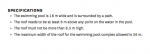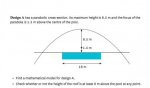I have worked out the equation for the parabola:

x^2 = -28(y-8.5)

However, I am puzzled on how to move on from that point onwards.
Again, it's another study I am stuck on!
Unfortunately I was only given one example on this particular equation (especially a negative quadratic equation), but it doesn't suit the problem I am trying to solve on this one.
Here is that example: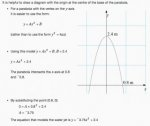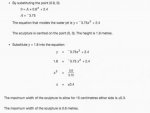I am unsure as to what I should do next. I am familiar with factorising and squaring but only worked on normal parabolas that already have their equations given. (The canvas is blank on this round!)
I feel that I need to substitute 6 metres somehow to give me an answer. I was trying to find a way to calculate everything that I have learnt but it doesn't seem to give me the answer I am looking for.
Trying not to rely too much on the desmos calculator but maybe I might need to for an answer.
Who knows? Would love your input on this. Cheers! :smile:

Jomo

Elite Member
Hi thereWould like a little help on this problem here if thats ok?

View attachment 10838 View attachment 10839

I have worked out the equation for the parabola:

x^2 = -28(y-8.5)

However, I am puzzled on how to move on from that point onwards.
Again, it's another study I am stuck on!
Unfortunately I was only given one example on this particular equation (especially a negative quadratic equation), but it doesn't suit the problem I am trying to solve on this one.
Here is that example:

View attachment 10843
View attachment 10842View attachment 10841

I am unsure as to what I should do next. I am familiar with factorising and squaring but only worked on normal parabolas that already have their equations given. (The canvas is blank on this round!)
I feel that I need to substitute 6 metres somehow to give me an answer. I was trying to find a way to calculate everything that I have learnt but it doesn't seem to give me the answer I am looking for.
Trying not to rely too much on the desmos calculator but maybe I might need to for an answer.
Who knows? Would love your input on this. Cheers! :smile:
OK, so you think that you have your equation. What exactly are you trying to do next and why are you struck?

JeffM

Elite Member
Hi thereWould like a little help on this problem here if thats ok?

View attachment 10838 View attachment 10839

I have worked out the equation for the parabola:

x^2 = -28(y-8.5)

However, I am puzzled on how to move on from that point onwards.
Again, it's another study I am stuck on!
Unfortunately I was only given one example on this particular equation (especially a negative quadratic equation), but it doesn't suit the problem I am trying to solve on this one.
Here is that example:

View attachment 10843
View attachment 10842View attachment 10841

I am unsure as to what I should do next. I am familiar with factorising and squaring but only worked on normal parabolas that already have their equations given. (The canvas is blank on this round!)
I feel that I need to substitute 6 metres somehow to give me an answer. I was trying to find a way to calculate everything that I have learnt but it doesn't seem to give me the answer I am looking for.
Trying not to rely too much on the desmos calculator but maybe I might need to for an answer.
Who knows? Would love your input on this. Cheers! :smile:
I have not checked your equation, but assuming it is correct and assuming that the equation is based on the horizontal line being the x-axis and the vertical line being the y-axis, at what points on the x-axis is the vertical distance between the pool and roof at a minimum?

If you know x, then how do you find the corresponding y?

What does that tell you?

LaaLa

New member
OK, so you think that you have your equation. What exactly are you trying to do next and why are you struck?
Hi Jomo,

I don't know what to do next with the equation x^2=-28(y-8.5)
I don't have the answer to the 6metre question. I don't know how to get there first.
I have been trying to figure it out for 2 days now. Everytime I try and test it on the desmos calculator, the numbers go out unevenly and doesn't seem to fit the equation of the parabola. Can someone give me the answer please and end my pain. I would appreciate it.

Last edited:

Dr.Peterson

Elite Member
I don't know what to do next with the equation x^2=-28(y-8.5)
I don't have the answer to the 6metre question. I don't know how to get there first.
I have been trying to figure it out for 2 days now. Everytime I try and test it on the desmos calculator, the numbers go out unevenly and doesn't seem to fit the equation of the parabola. Can someone give me the answer please and end my pain. I would appreciate it.
You may want to solve the equation for y, though that's not essential.

To check the 6 meter requirement, set x to 8 (one end of the pool) and find what y is. Is it at least 6?

You know the roof is no more than 8.5 m high anywhere.

You can check for the width being no more than 36 m either by solving for x when y = 0 (the base), or by setting x to 18 (half the maximum width) and seeing that y is negative (that is, it will have reached the ground before then).

But I don't see what trouble you could be having with Desmos. I pasted your equation in, and it's easy enough to see the answers (though you shouldn't be depending on that). Tell me what is going wrong when you use it. What do you see?

LaaLa

New member
You may want to solve the equation for y, though that's not essential.

To check the 6 meter requirement, set x to 8 (one end of the pool) and find what y is. Is it at least 6?

You know the roof is no more than 8.5 m high anywhere.

You can check for the width being no more than 36 m either by solving for x when y = 0 (the base), or by setting x to 18 (half the maximum width) and seeing that y is negative (that is, it will have reached the ground before then).

But I don't see what trouble you could be having with Desmos. I pasted your equation in, and it's easy enough to see the answers (though you shouldn't be depending on that). Tell me what is going wrong when you use it. What do you see?

I am not sure what you are meaning in in regards to finding 'y'. Are you meaning 'a'? The distance between the vertex and the focus? (8.5-1.5=7).

The equation x^2=-28(y-8.5) works on the desmos because I calculated it using the vertex and the focus. Hoping that it is the right equation unless someone kindly corrects me!
Notice the line I placed at 6 metres...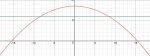When I talk about having problems with the desmos, I am trying to make the equation by using the technique to what I have been learning (from the one example I had supplied). When the equation is made however, I then get stuck and don't know where to go from then onwards.
The form used in the example is:
y= ax^2 + b
a = 18m (which is half of the base measurement)
b = 8.5m (vertex)

put it together...
y= 18^2 + 8.5

now if I work it out the way the example shows...
18^2 = 324
8.5/324 = 0.0262

to make sure this is correct...
-0.0262 x 18^2 = -8.5

Now if I put this on desmos alongside the other equation and the 6 metre line. This is what it looks like: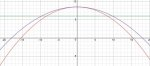Now I see they look slightly different to each other; the first equation was based on the vertex and focus which has no base measurement, whereas the 2nd equation does.

What is confusing me is that this equation is based on the measurements given from a design for building a roof. And when it comes to numbers and construction, most of the numbers are usually rounded. I tried to do the same when it came to this particular equation that I'm confused on. when I was in the middle of working out the second equation this is what happened:

The form used in the example is:
y= ax^2 + b
a = 18m (which is half of the base measurement)
b = 8.5m (vertex)

put it together...
y= 18^2 + 8.5

now if I work it out the way the example shows...
18^2 = 324

8.5/324 = 0.0262
= 0.03 (3s.f)

to make sure this is correct...
-0.03 x 18^2 = -9.72

Okay that didn't work, even though it's 0.01 difference. I will try it a different way:

18^2 = 324[/I]
8.5/324 = 0.0262
= 0.026

to make sure this is correct...
-0.026 x 18^2 = -8.424

Okay that is slightly 1 cm off.

The only option I see is to stay with the first set of numbers.

I would really like to use more than one option in regards to answering these questions without relying on using the desmos to show me the answers. It would be easier if I just give all the points and measurements by looking at the graph but this is about using the forms and methods I have already learnt to find the answer.

I don't know HOW to find out whether or not the height of the roof is at least 6 m above the pool at any point or whether or not the width of the pool roof is less the 36 metres.

If I make an equation for 6m height and 18m width of the pool, this is what it looks like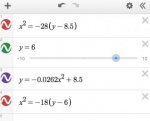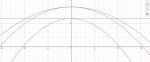I have questions!!
what point from the pool is it actually saying?

at least 6 m above the pool at any point.
at least 6 m above any point on the water in the pool.

Now it said "any point on the water...." does that mean vertically at any point or diagonally as well? What about horizontally??
Does that mean I have to add 6m to each side of the pool to get a safe answer??

How can I work this out? I am stuck and I have no idea what to do.

Dr.Peterson

Elite Member
I am not sure what you are meaning in in regards to finding 'y'. Are you meaning 'a'? The distance between the vertex and the focus? (8.5-1.5=7).
I meant exactly what I said: solve the equation for y! If I gave you the equation x^2=-28(y-8.5) and asked you to solve for y, what would you do? (Some people call it "making y the subject".)

If you don't do that, there will just be a little more work in other steps, but it is not essential, as I said. At some point, you have to find the value of y (the height of the roof) at the ends of the pool.

The equation x^2=-28(y-8.5) works on the desmos because I calculated it using the vertex and the focus. Hoping that it is the right equation unless someone kindly corrects me!
Yes, the equation is correct. Since they gave you the focus, what you did is exactly what is needed. Not all problems give the same information.

Notice the line I placed at 6 metres...
View attachment 10847View attachment 10848
Before we move on to what you say about this, do you see in the graph the answers to the questions? Is it wider than 36 meters at the base? Is it higher than 6 meters everywhere above the pool? This will not be the ultimate way you are supposed to answer those questions, which can be done without graphing, but the graph does help in understanding the questions.

When I talk about having problems with the desmos, I am trying to make the equation by using the technique to what I have been learning (from the one example I had supplied). When the equation is made however, I then get stuck and don't know where to go from then onwards.
The form used in the example is:
y= ax^2 + b
a = 18m (which is half of the base measurement)
b = 8.5m (vertex)

put it together...
y= 18^2 + 8.5
Why would "a" be half of the base measurement? The example you think you are following is entirely different (it doesn't mention the focus), and it doesn't set a to half the base width, but instead replaces x with that number and solves for a.

But you didn't even do what you say you're doing; you replaced "ax^2" with "18^2", not with 18x^2.

now if I work it out the way the example shows...
18^2 = 324
8.5/324 = 0.0262

to make sure this is correct...
-0.0262 x 18^2 = -8.5

Now if I put this on desmos alongside the other equation and the 6 metre line. This is what it looks like:
View attachment 10846View attachment 10849

Now I see they look slightly different to each other; the first equation was based on the vertex and focus which has no base measurement, whereas the 2nd equation does.
This formula is wrong, so they shouldn't look the same.

What is confusing me is that this equation is based on the measurements given from a design for building a roof. And when it comes to numbers and construction, most of the numbers are usually rounded. I tried to do the same when it came to this particular equation that I'm confused on. when I was in the middle of working out the second equation this is what happened:

The form used in the example is:
y= ax^2 + b
a = 18m (which is half of the base measurement)
b = 8.5m (vertex)

put it together...
y= 18^2 + 8.5

now if I work it out the way the example shows...
18^2 = 324

8.5/324 = 0.0262
= 0.03 (3s.f)

to make sure this is correct...
-0.03 x 18^2 = -9.72

Okay that didn't work, even though it's 0.01 difference. I will try it a different way:

18^2 = 324[/I]
8.5/324 = 0.0262
= 0.026

to make sure this is correct...
-0.026 x 18^2 = -8.424

Okay that is slightly 1 cm off.

The only option I see is to stay with the first set of numbers.
Again, this didn't work because the method is inappropriate for this problem, and you didn't follow it correctly anyway. Go with what you did correctly.

I would really like to use more than one option in regards to answering these questions without relying on using the desmos to show me the answers. It would be easier if I just give all the points and measurements by looking at the graph but this is about using the forms and methods I have already learnt to find the answer.

I don't know HOW to find out whether or not the height of the roof is at least 6 m above the pool at any point or whether or not the width of the pool roof is less the 36 metres.

If I make an equation for 6m height and 18m width of the pool, this is what it looks like
View attachment 10850View attachment 10851

I have questions!!
what point from the pool is it actually saying?

at least 6 m above the pool at any point.
at least 6 m above any point on the water in the pool.

Now it said "any point on the water...." does that mean vertically at any point or diagonally as well? What about horizontally??
Does that mean I have to add 6m to each side of the pool to get a safe answer??

How can I work this out? I am stuck and I have no idea what to do.
I think I told you what to do. Did you try following my suggestions?

The height above the pool at any point means the value of y for any x within the pool (that is, between -9 and 9). As you can see from the graph, or just know about quadratic equations with negative leading coefficient, y decreases as you move away from the axis, so you only need to check the value of y when x = 9 (or -9), which are the locations of the ends of the pool.

Height is always measured vertically. As I said, it's what y means in this problem.

Try again, and just show me the work of using your good equation to answer the questions, so we can keep things simple.

JeffM

Elite Member
It seems to me that you are not asking yourself what things mean. This problem is not hard once you ask about meaning rather than tools. A first step in grasping what the problem means is to write down brief definitions for your variables.

$$\displaystyle x = \text {horizontal distance from middle line of pool.}$$

$$\displaystyle y = \text {vertical distance from pool level to roof.}.$$

Why do we use vertical distance? Because if the vertical distance from a point on the pool's surface is 6m, the distance in any other direction is more than 6m.

Your question is about roof height, y, but your equation does not give you y. So the first tool that is going to be handy is to re-arrange the equation so that it gives you y. As Dr. P says, you do not have to do this, but if you are looking for y, it is obviously going to be extremely convenient to have an equation in the form y = f(x). Show us what you get from putting the equation into a form that is most useful for solving this problem.

Now we think again. We need to know whether the vertical distance between pool surface and roof falls below some number. Consider your diagram. Where is that distance at a minimum? Is it at the midline. Obviously not. The distance is at a minimum at the edges of the pool because the parabola is always falling from its vertex (peak). Please tell us what is the value of x at the edge of the pool?

Once you know what value to assign to x, what does your equation say is the value of y?

How does this compare to 6?

Last edited:

LaaLa

New member
I meant exactly what I said: solve the equation for y! If I gave you the equation x^2=-28(y-8.5) and asked you to solve for y, what would you do? (Some people call it "making y the subject".)
Hi Dr.Peterson, thanks for getting back to me. I appreciate the time you are taking to help me out.
So far on parabolas I have learnt how to identify them using the key information (vertex, focus, centre, directrix and latus rectum ends) and drawing them onto graphs. I have also learnt about parametric equations, implicit differentiation, gradients, tangents, normals and intersections.
Coming across this exercise in the study has put me to a halt. I am unfamiliar with this type of parabola and problem. The only exercise I have done was this one:What do you mean when you say 'find the y'? I have not come across an exercise like that in this study before. I have with ellipses and circles but not parabolas. Do you mean I must differentiate the parabola equation?

Why would "a" be half of the base measurement? The example you think you are following is entirely different (it doesn't mention the focus), and it doesn't set a to half the base width, but instead replaces x with that number and solves for a.

I don't understand when you say this example is entirely different. Are you saying I should be using a different technique??
The example is the only method I have learnt in regards to problem solving. I will explain how I got the idea...

EXAMPLE states:
- HEIGHT of 2.4metres
- WIDTH of 1.6metres

If you divide the width by half you get 0.8metres
It was also stated in the example that the parabola intersects the x-axis at (8,0) and (-8,0).
0.8metres is exactly what I got by dividing the width.

The form used: y= ax^2+b
for 'a' they used 0.8metres (width from centre to end of parabola)
for 'b' they used 2.4metres (height from centre to top of parabola)

If I use the same form but give different figures using the design I am working on:
- HEIGHT of 8.5metres
- WIDTH of 36metres
Divide the width by half you get 18metres
It was also stated that whether or not the width of the pool roof is less the 36 metres - I wouldn't have a clue what the width is, since 36metres was mentioned in the first place, I assume that number is what the width is.

a= 18metres
b= 8.5metres

But you didn't even do what you say you're doing; you replaced "ax^2" with "18^2", not with 18x^2.
Can you explain please how it makes any difference adding an 'x' in there?
I shall correct it then...

y= 18x^2 + 8.5

sorry if this has confused you, but I really need an explanation for everything.

The height above the pool at any point means the value of y for any x within the pool (that is, between -9 and 9). As you can see from the graph, or just know about quadratic equations with negative leading coefficient, y decreases as you move away from the axis, so you only need to check the value of y when x = 9 (or -9), which are the locations of the ends of the pool.
THANK YOU!! That makes total sense! I wondered maybe those particular points could be used since it was the only information given other than the focus and vertex. I just don't know how to solve this now.
I don't know what I am doing so I went online to use the symbolab calculator which hopefully has given me a correct answer:

Y^2=-28(y-8.5)
EXPAND
(x^2)(-28y)((-28x-8.5=238)
x^2=-28y+238

SIMPLIFY
x^2= 28-238
x^2= 210
x^2= √210 = 14.491
x= 14.5

I am hoping this is the answer to the distance from the centre to the x-intercept either side?
So if I multiply it by 2, the total distance is 29metres?

I am still not sure where to go from there. I feel that somehow I need to substitute Point (9,0) into the equation but I don't know how to do it.
Can someone just please make it clear to me because I really don't know what to do. I'm just guessing everything now and I don't know where I'm going and I would like some guidance for the answer so in the future I can remember and use it again. Please, thank you

Last edited:

Dr.Peterson

Elite Member
Hi Dr.Peterson, thanks for getting back to me. I appreciate the time you are taking to help me out.
So far on parabolas I have learnt how to identify them using the key information (vertex, focus, centre, directrix and latus rectum ends) and drawing them onto graphs. I have also learnt about parametric equations, implicit differentiation, gradients, tangents, normals and intersections.
Coming across this exercise in the study has put me to a halt. I am unfamiliar with this type of parabola and problem.

What do you mean when you say 'find the y'? I have not come across an exercise like that in this study before. I have with ellipses and circles but not parabolas. Do you mean I must differentiate the parabola equation?
Surely you know how to solve an equation for a single variable!

What I mean is to rewrite "x^2 = -28(y-8.5)" as "y = ...". You can divide both sides by -28, then add 8.5 to both sides. There is nothing advanced about this.

I don't understand when you say this example is entirely different. Are you saying I should be using a different technique??
The example is the only method I have learnt in regards to problem solving. I will explain how I got the idea...

EXAMPLE states:
- HEIGHT of 2.4metres
- WIDTH of 1.6metres

If you divide the width by half you get 0.8metres
It was also stated in the example that the parabola intersects the x-axis at (8,0) and (-8,0).
0.8metres is exactly what I got by dividing the width.

The form used: y= ax^2+b
for 'a' they used 0.8metres (width from centre to end of parabola)
for 'b' they used 2.4metres (height from centre to top of parabola)

If I use the same form but give different figures using the design I am working on:
- HEIGHT of 8.5metres
- WIDTH of 36metres
Divide the width by half you get 18metres
It was also stated that whether or not the width of the pool roof is less the 36 metres - I wouldn't have a clue what the width is, since 36metres was mentioned in the first place, I assume that number is what the width is.

a= 18metres
b= 8.5metres
I meant what I said: that example doesn't use the focus, so you can't do the same steps.

But I also meant it when I said your equation was correct. Forget this example!!! Just think about the problem you're working on. You have a correct equation; now just answer the questions about it.

Can you explain please how it makes any difference adding an 'x' in there?
I shall correct it then...

y= 18x^2 + 8.5

sorry if this has confused you, but I really need an explanation for everything.
Surely you know that 18^2 and 18x^2 are not the same thing! Without the x, it's just a constant.

THANK YOU!! That makes total sense! I wondered maybe those particular points could be used since it was the only information given other than the focus and vertex. I just don't know how to solve this now.
I don't know what I am doing so I went online to use the symbolab calculator which hopefully has given me a correct answer:

Y^2=-28(y-8.5)
EXPAND
(x^2)(-28y)((-28x-8.5=238)
x^2=-28y+238

SIMPLIFY
x^2= 28-238
x^2= 210
x^2= √210 = 14.491
x= 14.5

I am hoping this is the answer to the distance from the centre to the x-intercept either side?
So if I multiply it by 2, the total distance is 29metres?

I am still not sure where to go from there. I feel that somehow I need to substitute Point (9,0) into the equation but I don't know how to do it.
Can someone just please make it clear to me because I really don't know what to do. I'm just guessing everything now and I don't know where I'm going and I would like some guidance for the answer so in the future I can remember and use it again. Please, thank you
I'm not sure what you think you were doing there, but you seem to have solved for x when y = -1. That's irrelevant.

Please think for yourself. You don't need a computer to do anything for you. This issue here is understanding how questions are related to the equation, which the computer will not do for you.

Answering these long posts is very difficult. What I want from you is a minimal response, so I can answer just one question at a time.

Here's the first question. You have your equation, x^2 = -28(y - 8.5). Although you never stated it explicitly, x means the distance from the center of the pool and y is the height above ground. What is the height above the point x=9, which is at one end of the pool? That is, what is y, when x is 9?

Just answer that one question; don't tell me anything else yet. That will help us stay focused.

LaaLa

New member
Surely you know how to solve an equation for a single variable!
Hi Dr.Peterson,
no I do not know how to do this. I can't recall it through my studies. In fact, I have no idea what a single variable is.

What I mean is to rewrite "x^2 = -28(y-8.5)" as "y = ...". You can divide both sides by -28, then add 8.5 to both sides. There is nothing advanced about this.
I still don't understand what you mean. I don't think I have learnt this technique.

Surely you know that 18^2 and 18x^2 are not the same thing! Without the x, it's just a constant.
No I don't know!! I don't know the difference. I am more of a practical learner. Give me a pattern and I will learn it and use it. Give me a story and I don't seem to understand it unless it is shown to me. If you can give me an example of how things are worked out it might be easier for me to understand rather than trying to put it into words. All these terms are confusing me.

I'm not sure what you think you were doing there, but you seem to have solved for x when y = -1. That's irrelevant.
I have no idea what I am doing either! I am doing what I said I was = guessing! And I was hoping I got the correct answer, but unfortunately not.

Please think for yourself. You don't need a computer to do anything for you. This issue here is understanding how questions are related to the equation, which the computer will not do for you.
I understand what it is they are looking for, but I can't figure out the method for giving the answers without having to look at the graph. I want to use every single method, but I don't know all of them, in fact any of them (except using the graph, differentiations, gradients, tangents, intersections and the discriminant). I have been constantly searching online for methods. I believe the quality of thinking and how well you link this to the context will determine the overall grade of your learning. My study papers have constantly given me examples and answers but this time it is an exercise which I have to figure out by using the examples they have already previously given. What examples! The only example is the one I have shown you. I have looked through all my study papers 5 times now and haven't come across anything related to parabolas without the parabola already being complete with the key information.

Here's the first question. You have your equation, x^2 = -28(y - 8.5). Although you never stated it explicitly, x means the distance from the center of the pool and y is the height above ground. What is the height above the point x=9, which is at one end of the pool? That is, what is y, when x is 9?
For that equation x^2=-28(y-8.5) I had to do lots of research online and use symbolab calculator to get that answer! My study papers never mentioned anything about this type of equation! Which is why I used the example that I had shown in the previous post. I finally understand how it is done now but it took a long time to get to this stage.

I don't know how to work out what the height is above the points (±9,0) What method do you use?
I don't have a method to work this out.
Sorry if this is frustrating you, I have spent an entire week on this one exercise for 10 hours each day, I hope you understand how I feel!

Last edited:

Dr.Peterson

Elite Member
Hi Dr.Peterson,
no I do not know how to do this. I can't recall it through my studies. In fact, I have no idea what a single variable is.

I still don't understand what you mean. I don't think I have learnt this technique.

No I don't know!! I don't know the difference. I am more of a practical learner. Give me a pattern and I will learn it and use it. Give me a story and I don't seem to understand it unless it is shown to me. If you can give me an example of how things are worked out it might be easier for me to understand rather than trying to put it into words. All these terms are confusing me.

I have no idea what I am doing either! I am doing what I said I was = guessing! And I was hoping I got the correct answer, but unfortunately not.

I understand what it is they are looking for, but I can't figure out the method for giving the answers without having to look at the graph. I want to use every single method, but I don't know all of them, in fact any of them (except using the graph, differentiations, gradients, tangents, intersections and the discriminant). I have been constantly searching online for methods. I believe the quality of thinking and how well you link this to the context will determine the overall grade of your learning. My study papers have constantly given me examples and answers but this time it is an exercise which I have to figure out by using the examples they have already previously given. What examples! The only example is the one I have shown you. I have looked through all my study papers 5 times now and haven't come across anything related to parabolas without the parabola already being complete with the key information.

For that equation x^2=-28(y-8.5) I had to do lots of research online and use symbolab calculator to get that answer! My study papers never mentioned anything about this type of equation! Which is why I used the example that I had shown in the previous post. I finally understand how it is done now but it took a long time to get to this stage.

I don't know how to work out what the height is above the points (±9,0) What method do you use?
I don't have a method to work this out.
Sorry if this is frustrating you, I have spent an entire week on this one exercise for 10 hours each day, I hope you understand how I feel!
I guess we have to start at the beginning.

Can you solve this equation for x?

5x - 2 = 18

We'll move on from there until you know how to solve the kind of equation you have for a variable.

If you've been moving through math without paying attention to meanings and the reason for what you do, then you are going to get stuck somewhere. Maybe this is where you have to go back and relearn things with understanding.

JeffM

Elite Member
Hi Dr.Peterson,
no I do not know how to do this. I can't recall it through my studies. In fact, I have no idea what a single variable is.
Are you seriously telling us that you do not know to solve

$$\displaystyle 3x + 4 = 5(x - 6).$$

That is an equation in one unknown, namely x, (sometimes inaccurately called a variable). If you cannot solve that to find out what number is represented by x, you need to study basic algebra.

I still don't understand what you mean. I don't think I have learnt this technique.
We frequently have equations in 2 or more variables. For example,

$$\displaystyle c = \dfrac{5}{9} * (f - 32).$$

The variables are c and f. They are called "variables" because we can replace them with various numbers. If we replace f with 212, we get

$$\displaystyle c = \dfrac{5}{9} * (212 - 32) = \dfrac{5}{9} * 180 = 100.$$

That is the formula for converting temperatures in Fahrenheit to centigrade. But suppose we want the opposite formula, to translate centigrade into Fahrenheit. We solve for f in terms of c.

$$\displaystyle c = \dfrac{5}{9} * (f - 32) \implies$$

$$\displaystyle \dfrac{9}{5} * c = \dfrac{9}{5} * \dfrac{5}{9} (f - 32) \implies$$

$$\displaystyle \dfrac{9}{5} * c = f - 32 \implies$$

$$\displaystyle f \ = \ 32 + \dfrac{9}{5} * c.$$

Basic, basic algebra.

No I don't know!! I don't know the difference. I am more of a practical learner. Give me a pattern and I will learn it and use it. Give me a story and I don't seem to understand it unless it is shown to me. If you can give me an example of how things are worked out it might be easier for me to understand rather than trying to put it into words. All these terms are confusing me.
$$\displaystyle 18x^2$$ means that you multiply the number x by itself and then multiply that product by 18.

$$\displaystyle 18^2$$ means that you multiply 18 by itself.

If you do not understand algebraic notation, you will find it virtually impossible to work with algebraic formulas and equations.

I understand what it is they are looking for, but I can't figure out the method for giving the answers without having to look at the graph. I want to use every single method, but I don't know all of them, in fact any of them (except using the graph, differentiations, gradients, tangents, intersections and the discriminant).
How in the world can you do differentiation without knowing any algebra?

I don't know how to work out what the height is above the points (±9,0) What method do you use?
I don't have a method to work this out.
Have you read any of my posts? What does y mean?

Last edited by a moderator:

LaaLa

New member
I guess we have to start at the beginning.

Can you solve this equation for x?

5x - 2 = 18

We'll move on from there until you know how to solve the kind of equation you have for a variable.

If you've been moving through math without paying attention to meanings and the reason for what you do, then you are going to get stuck somewhere. Maybe this is where you have to go back and relearn things with understanding.
I don't know what I am doing but I'll give it a go...

5x - 2 = 18

5x - 2 - 18
5x = 2 - 18
5x = 16
x = 16/5
x = 3.2

I hope I got that right? I have no idea what I done there, but it's a pattern I remember.

Dr.Peterson

Elite Member
I don't know what I am doing but I'll give it a go...

5x - 2 = 18

5x - 2 - 18
5x = 2 - 18
5x = 16
x = 16/5
x = 3.2

I hope I got that right? I have no idea what I done there, but it's a pattern I remember.
The next step is to practice checking your work. There are at least two errors in there. Can you find and fix them?

Then check your result, by putting it into the original equation to see if it's correct.

LaaLa

New member
The next step is to practice checking your work. There are at least two errors in there. Can you find and fix them?

Then check your result, by putting it into the original equation to see if it's correct.
Oh wow I think I get what you mean!!

5x - 2 = 18

5x = 18 + 2
5x = 20
x = 5/20
x = 4

And it's correct because
5 x 4 = 20 - 2 = 18Denis

Senior Member
5x = 20
x = 5/20 ********
x = 4
******** should be x = 20/5
But you got the right answerDr.Peterson

Elite Member
Oh wow I think I get what you mean!!

5x - 2 = 18

5x = 18 + 2
5x = 20
x = 5/20
x = 4

And it's correct because
5 x 4 = 20 - 2 = 18Good, apart from writing the division backward (though what you meant was right).

This illustrates something I tell students all the time: in order to do math well, you have to:

1. Think.
2. Write what you thought.
3. Think about what you wrote.
4. Fix it!
Rushing through work without thinking about it is a waste of time; and rushing off thinking you got it right without checking wastes additional time. And you can't check what you did if you haven't written out the steps. Every time I write a line, I check that it is what I meant to do.

Now, let's take the next step.

Can you solve this equation for x?

5x - 2 = y

LaaLa

New member
Can you solve this equation for x?

5x - 2 = y
hmmm, this is slightly trickier as it includes a y which I am not familiar with.
I'm not a hundred percent sure so I will give two estimates.

#1
5x - 2 = y
5(x-2)=y
5x - 10 = y
5x = y + 10
y= 5x + 10

#2
5x - 2 = y
5x = y+2
x = y+2 /5

I have a feeling this might be wrong. To me it looks a lot better on the first guess. I can never be sure though.
Is the first one x=-2?? I just noticed that 5 x -2 = -10, if you use the other 10 that is positive, they cancel each other out.
To me it makes more sense that way

Last edited:

HallsofIvy

Elite Member
hmmm, this is slightly trickier as it includes a y which I am not familiar with.
I'm not a hundred percent sure so I will give two estimates.

#1
5x - 2 = y
5(x-2)=y
No, you cannot "factor out a 5" and still have "2" left alone

5x - 10 = y
And now multiplied the "5" back in and have 10 instead of 2? Surely you understand that "5x- 10" is NOT the same as "5x- 2"!

5x = y + 10
y= 5x + 1

#2
5x - 2 = y
5x = y+2
x = y+2 /5

I have a feeling this might be wrong. To me it looks a lot better on the first guess. I can never be sure though.
Is the first one x=-2?? I just noticed that 5 x -2 = -10, if you use the other 10 that is positive, they cancel each other out.
To me it makes more sense that way
No, the first makes no sense at all! The second is correct. There is no "10" in this problem!